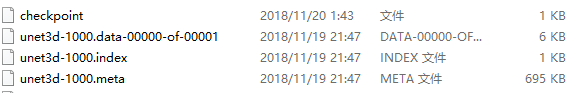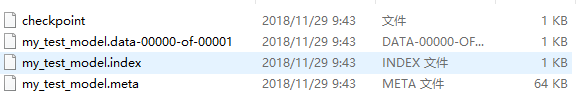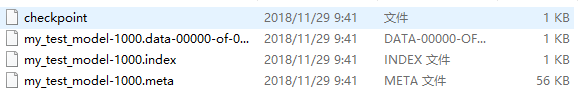1、什麼是 tensorflow 模型

tensorflow 模型包括：已訓練並優化的權重引數，網路結構和 graph。

tensorflow 模型檔案包括兩大塊：

• meta graph ：序列化緩衝檔案，儲存完整的網路結構，graph ，即 all variables, operations, collections 等，副檔名是 .meta
• checkpoint file：二進位制檔案，包括 weights, biases, gradients 和 all the other variables，副檔名為 .ckpt 。但是從0.11版本開始，就不是單獨的 .ckpt 檔案了，而是有兩個檔案：
>>mymodel.data-00000-of-00001 #包括訓練變數，可從這個檔案開始繼續訓練
>>mymodel.index2、儲存 tensorflow 模型

tensorflow 中的變數只在會話 session 中存在，所以需要在 saver 物件上呼叫 save 方法，將模型儲存在會話中。

#模型的儲存
import tensorflow as tf
import os

w1 = tf.Variable(tf.random_normal(shape=), name='w1')
w2 = tf.Variable(tf.random_normal(shape=), name='w2')
saver = tf.train.Saver() #可指定需要儲存的tensor，不指定則全部儲存
with tf.Session() as sess:
#sess = tf.Session()
sess.run(tf.global_variables_initializer())
#建立儲存模型的資料夾
if not os.path.exists('my_model'):
os.mkdir('./my_model')
saver.save(sess, './my_model/my_test_model')

#可通過設定saver.save()的引數指定儲存哪一步的模型
saver.save(sess, './my_model/my_test_model', global_step=1000) #儲存1000步的模型

# This will save following files in Tensorflow v >= 0.11
# my_test_model.data-00000-of-00001
# my_test_model.index
# my_test_model.meta
# checkpoint1000步的模型，會在 my_test_model 後 append ‘-1000’.meta 儲存的是網路結構，訓練過程中不改變網路結果，儲存一次即可，可使用如下語句：

saver.save(sess, './my_model/my_test_model', global_step=step, write_meta_graph=False)

#saves a model every 2 hours and maximum 4 latest models are saved.
saver = tf.train.Saver(max_to_keep=4, keep_checkpoint_every_n_hours=2)

#將需要儲存的變數以列表形式新增在saver中？自己的理解~確實是這個語句
saver = tf.train.Saver([w1, w2])

3、載入預訓練模型

• 使用如下語句載入網路結構：
saver = tf.train.import_meta_graph('./my_model/my_test_model.meta')
• 使用如下語句載入引數：
import tensorflow as tf
with tf.Session() as sess:
new_saver = tf.train.import_meta_graph('./my_model/my_test_model.meta') #載入網路結構
new_saver.restore(sess, tf.train.latest_checkpoint('./my_model')) #載入最近一次儲存的ckpt
#初始化引數
sess.run(tf.global_variables_initializer())
print(sess.run('w1:0'))
#返回：INFO:tensorflow:Restoring parameters from ./my_model\my_test_model
[ 0.35064858  2.87996149]

https://blog.csdn.net/liuxiao214/article/details/79048136